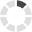### Site InformationLoading... Please wait...

# Determine Electric Cost

How to Calculate Electric Costs for Pumps and Aerators

Be careful when comparing pumps. Always compare GPH (gallons per hour) vs. amps draw. To calculate the operating cost of an electric motor use the following formula:

Amps x volts (115 or 230 volts) = watts x hours per day of operation ÷ 1000 x cost per kilowatt hour.

For example: Say you are looking at one of our aerators and you read that it draws 1 amp of electricity and it uses 115 volts. This aerator (as well as others) should run 24 hours a day.  What we need to do to figure out the total cost is to first get out our electric bill and find what we pay per kilowatt hour.

When viewing your electric bill, find where it states the kilowatt or kwh. You'll find some numbers something like this: 240kwh @ .9704 or .1003. The numbers will be different throughout the country as electric costs vary. Our electric costs is .1002 and I'll use that for this example. This .1002 means that it costs me 10 cents (use the first numbers after the decimal point) per kilowatt hour. Now, let's plug this information into our equation:

1amp x 115volt = watts x 24 hours per day ÷ 1000 x .10

This calculates to:
1 x 115 = 115 watts. 115 watts x 24 hours = 2760. 2760 ÷ 1000 = 2.76.
2.76 x .10 = .276 or nearly 28 cents per day to run that particular aerator (or anything electric, for that matter).

For months having 30 days, I multiply the .28cents per day x 30 days and I get \$8.40.  That is the price it will cost me to run that aerator 24 hours a day for 30 days.

Sometimes you will see a big difference in prices for comparable pumps throughout our site. This is not only due the individual features of the pumps but because of how energy efficient they are.

For example, I recently compared 2 different 3000 GPH pumps.  One cost \$215.89 and used 700 watts while another 3000 GPH pump sells for \$700.00 and only draws 260 amps.

The first pump would cost us \$50.40 per month to operate while the second pump would cost \$18.72 per month.  The first pump would therefore cost over 2 1/2 times per month to operate compared to the second pump. Granted, there is also a substantial difference in price (almost 2 1/2 times) between the two pumps but it definitely makes it worthwhile to know the operating cost of any product requiring electricity to operate it to determine if you can afford the change in your electric bill.